Question

I know some of the measured pH values are a little off due to error in...

I know some of the measured pH values are a little off due to error in the lab, but I need help filling out the “Ion Hydrolyzed” table and then the “Net Ionic Equation” part. I remember doing net ionic equations in General Chemistry I, bit don’t see how it applies here.

Thanks, in advance! (i will rate!)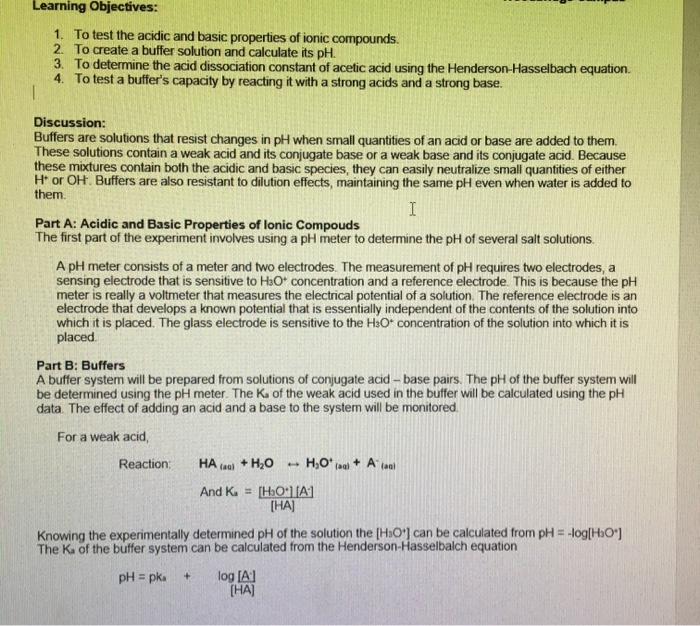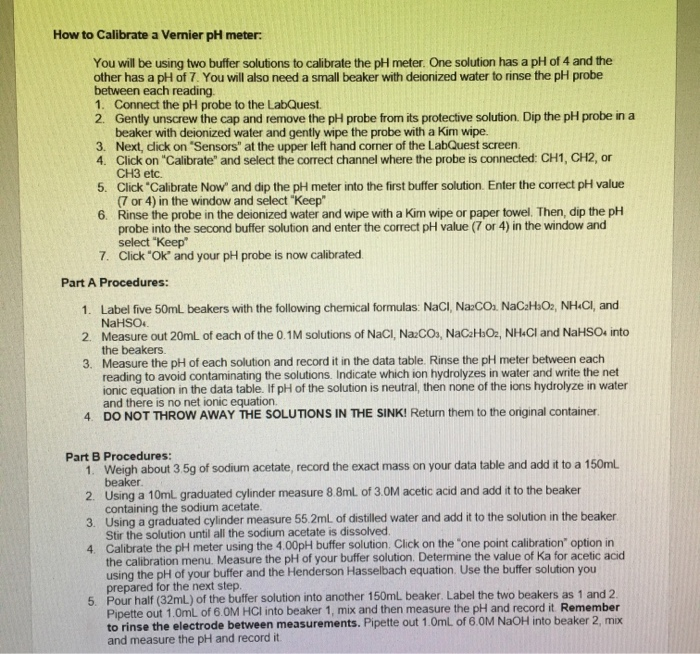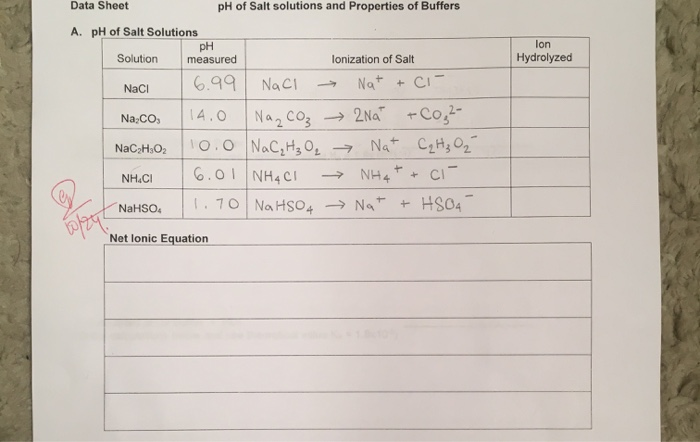Learning Objectives: 1. To test the acidic and basic properties of ionic compounds. 2. To create a buffer solution and calculate its pH. 3. To determine the acid dissociation constant of acetic acid using the Henderson-Hasselbach equation. 4. To test a buffer's capacity by reacting it with a strong acids and a strong base. Discussion: Buffers are solutions that resist changes in pH when small quantities of an acid or base are added to them. These solutions contain a weak acid and its conjugate base or a weak base and its conjugate acid. Because these mixtures contain both the acidic and basic species, they can easily neutralize small quantities of either Hi or OH. Buffers are also resistant to dilution effects, maintaining the same pH even when water is added to them. Part A: Acidic and Basic Properties of lonic Compouds The first part of the experiment involves using a pH meter to determine the pH of several salt solutions. A pH meter consists of a meter and two electrodes. The measurement of pH requires two electrodes, a sensing electrode that is sensitive to H3O+ concentration and a reference electrode. This is because the pH meter is really a voltmeter that measures the electrical potential of a solution. The reference electrode is an electrode that develops a known potential that is essentially independent of the contents of the solution into which it is placed. The glass electrode is sensitive to the H2O concentration of the solution into which it is placed. Part B: Buffers A buffer system will be prepared from solutions of conjugate acid-base pairs. The pH of the buffer system will be determined using the pH meter. The K of the weak acid used in the buffer will be calculated using the pH data. The effect of adding an acid and a base to the system will be monitored. For a weak acid, Reaction: HA (aul + H20 - H,O*raq + Alaal And K = [H3OA1 [HA] Knowing the experimentally determined pH of the solution the [H3O') can be calculated from pH = -log[H3O'] The K of the buffer system can be calculated from the Henderson-Hasselbalch equation pH = pk + log [A] [HA]
lon Hydrolyzed Data Sheet pH of Salt solutions and Properties of Buffers A. pH of Salt Solutions pH Solution measured lonization of Salt NaCl 16.99 NaCl Nat + ci- Na co, 14.0 Na₂CO₃ → 2N0 + CO²- NaC H302 110.0 NaC₂H₂O₂ → Nat C₂H₂ Oi NH.CI 6.01 NHCI → NH4+ + ci- NaHSO. 1.70 NaHSO4 → Na+ + HSO4- Net lonic Equation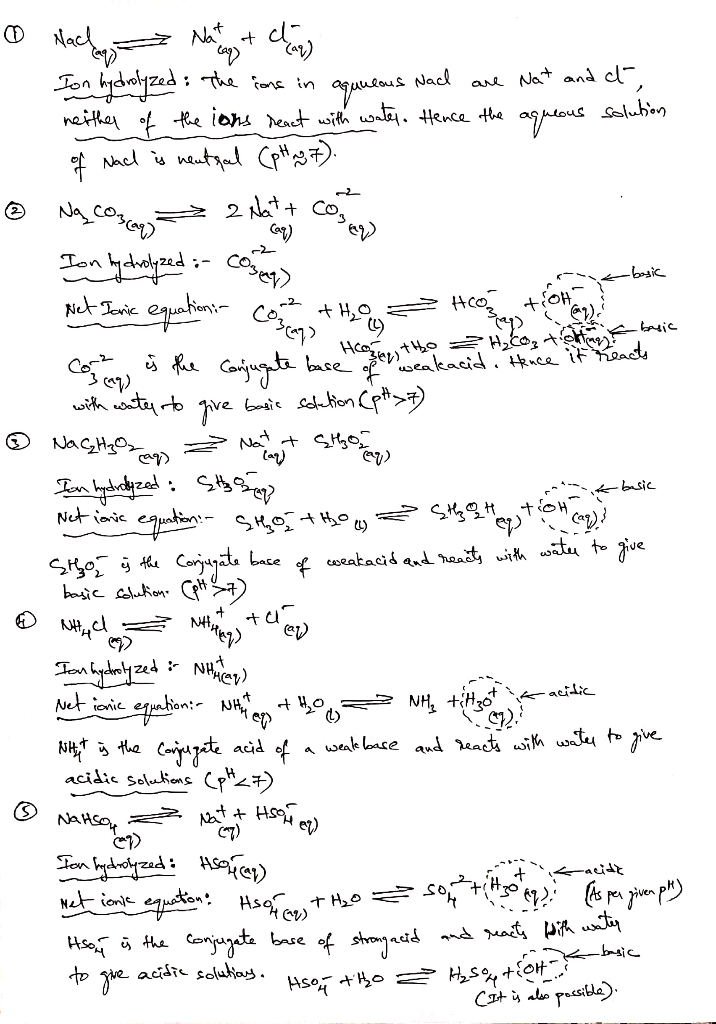Earn Coins

Coins can be redeemed for fabulous gifts.

Similar Homework Help Questions
• This is from a Study of Buffer Solutions and pH of Salt Solutions Lab. I calculated...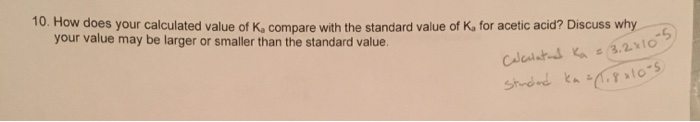This is from a Study of Buffer Solutions and pH of Salt Solutions Lab. I calculated Ka to be 3.2*10^-5. Why is my value larger than the standard value? Procedure: 10. How does your calculated value of Ka compare with the standard value of Ka for acetic acid? Discuss why your value may be larger or smaller than the standard value. Caleutats Ka 3.2x 10-5) Cyato-s Learning Objectives: 1. To test the acidic and basic properties of ionic compounds 2....

• I need help with the problem in the last photo.. I thought I’d post my lab...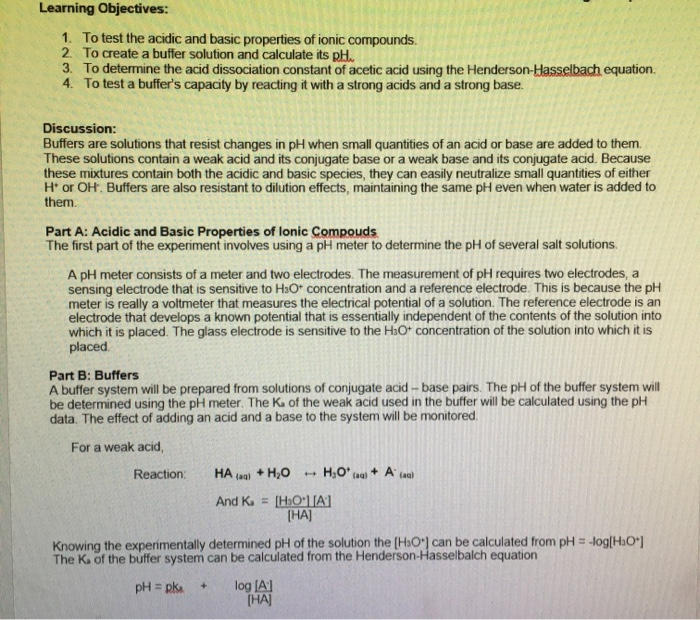I need help with the problem in the last photo.. I thought I’d post my lab explanation and data if that helps you get a better understanding, but it’s just the question at the end. I know I need to use the Henderson Hasselbach equation.. so... 4.70 = pKa + log( [acetate-ion] / [acetic-ion] ) and solve for pKa, then Ka.. but how do I find the concentrations to put in the log fraction? Thanks, in advance! Learning Objectives: 1....

• Properties of Buffers Lab. I need help with problems 7 & 8. They’re pretty similar, but...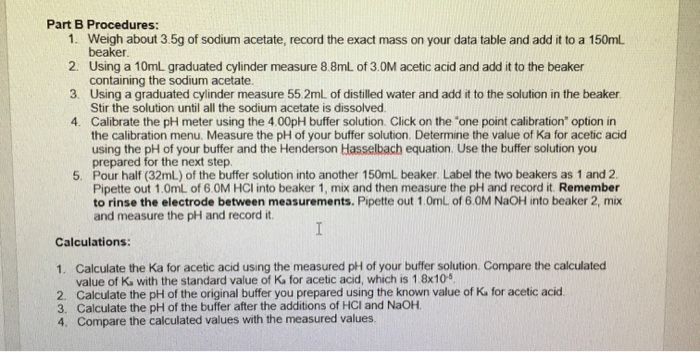Properties of Buffers Lab. I need help with problems 7 & 8. They’re pretty similar, but I’m confused on whether I’m doing them right. I need to calculate the moles of HCl and NaOH and then somehow insert them into the log fraction in the Henderson Hasselbach formula, is that right? Procedure (values are needed in procedure): Measured values during lab that I’ll be comparing #6/7/8 to: Questions I need help with: Part B Procedures: 1. Weigh about 3.5g of...

• F. pH of Buffer after the addition of the Strong Base 1. Calculate the expected pH...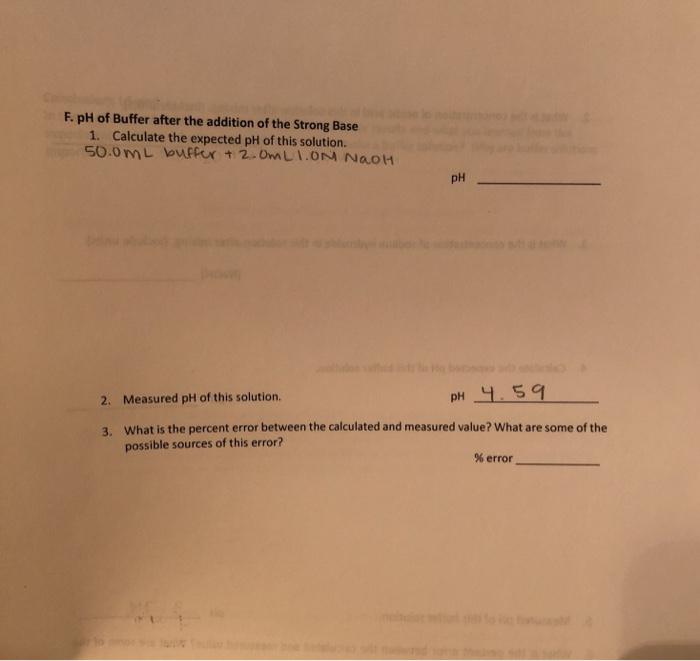F. pH of Buffer after the addition of the Strong Base 1. Calculate the expected pH of this solution. 50.0 mL buffer + 2.0mLION NAOH pH 2. Measured pH of this solution. pH 4.59 3. What is the percent error between the calculated and measured value? What are some of the possible sources of this error? % error pH of Buffer after the addition of the Strong Base: ix. Measure out 50.0 ml the buffer in part D and 2.0...

• how would you calculate the expected pH for solution 7, 8, 9, 10, and 11? (I...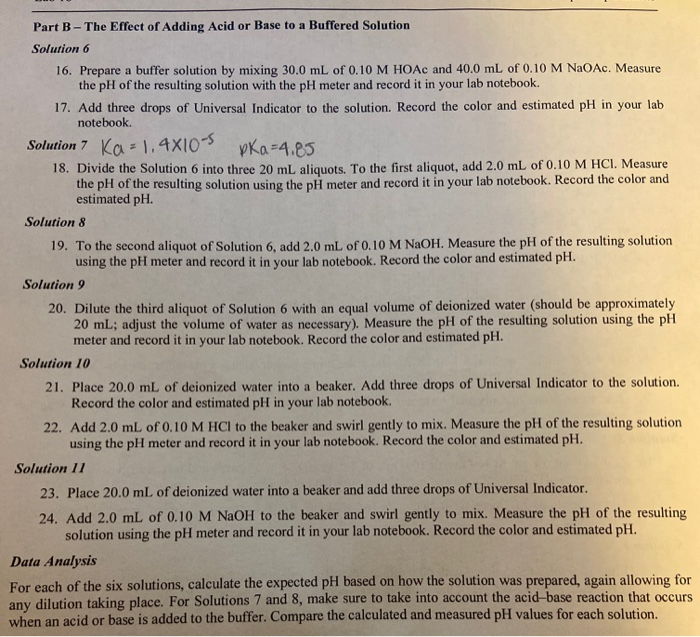how would you calculate the expected pH for solution 7, 8, 9, 10, and 11? (I am confused on how you calculate the dilutions for finding the expected pH too) Ka= 1.4x10^-5 pKa= 4.85 Part B - The Effect of Adding Acid or Base to a Buffered Solution Solution 6 16. Prepare a buffer solution by mixing 30.0 mL of 0.10 M HOAc and 40.0 mL of 0.10 M NaOAc. Measure the pH of the resulting solution with the pH...

• based on instructions please help me fill out chart Use appropriate safety precautions for working with...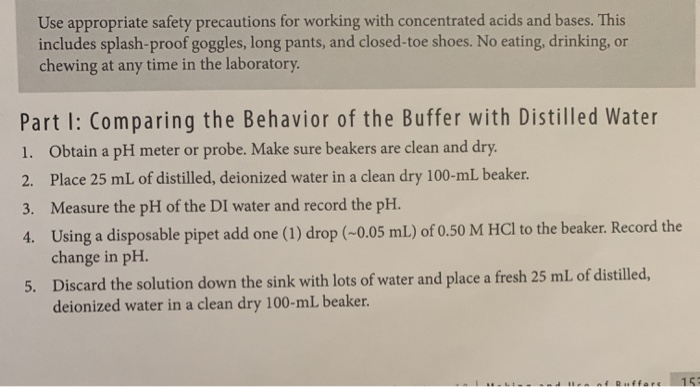based on instructions please help me fill out chart Use appropriate safety precautions for working with concentrated acids and bases. This includes splash-proof goggles, long pants, and closed-toe shoes. No eating, drinking, or chewing at any time in the laboratory. Part I: Comparing the Behavior of the Buffer with Distilled Water 1. Obtain a pH meter or probe. Make sure beakers are clean and dry. 2. Place 25 mL of distilled, deionized water in a clean dry 100-ml beaker. 3....

• Please help, I'm so confused!!!! This is due wednesday night!!! i'm gonna fail :(((( pH of...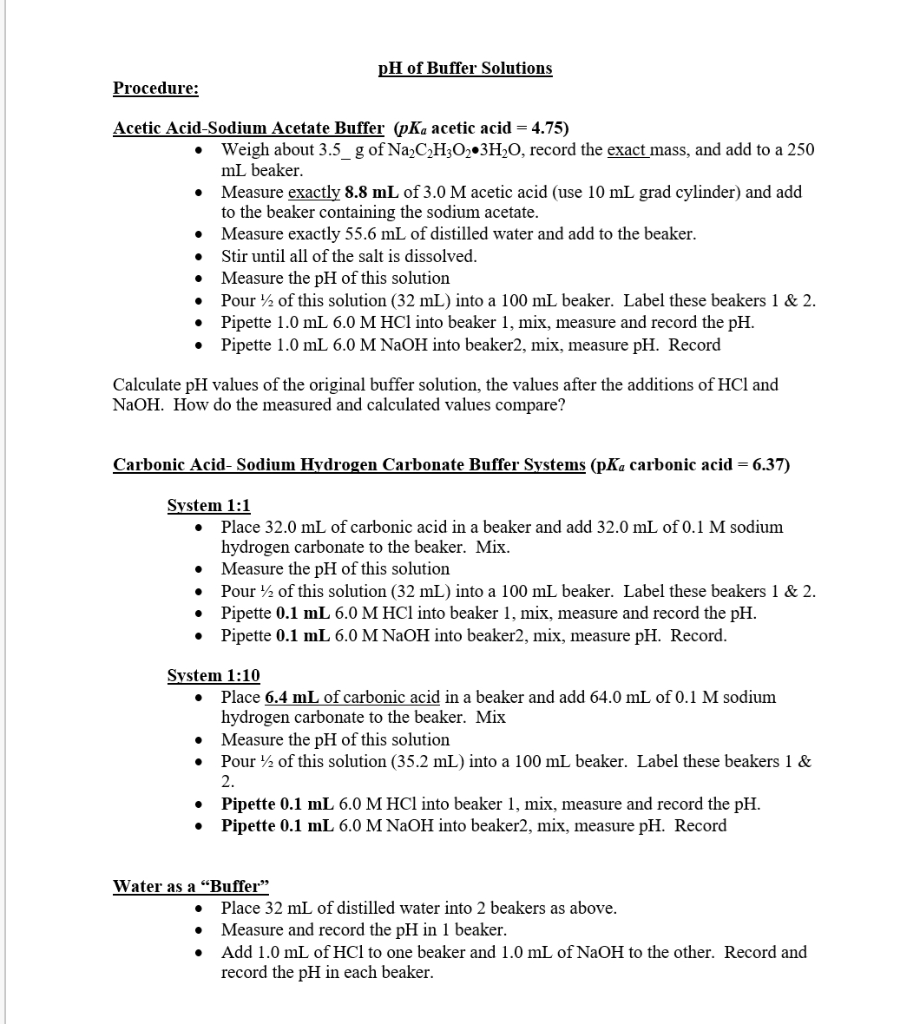Please help, I'm so confused!!!! This is due wednesday night!!! i'm gonna fail :(((( pH of Buffer Solutions Procedure: Acetic Acid-Sodium Acetate Buffer (pKa acetic acid = 4.75) Weigh about 3.5 g of Na2C2H302 3H2O, record the exact mass, and add to a 250 ml beaker. Measure exactly 8.8 mL of 3.0 M acetic acid (use 10 mL grad cylinder) and add to the beaker containing the sodium acetate. • Measure exactly 55.6 mL of distilled water and add to...

• Please answer why to #1 and answer #2 I posted the directions so it is easier...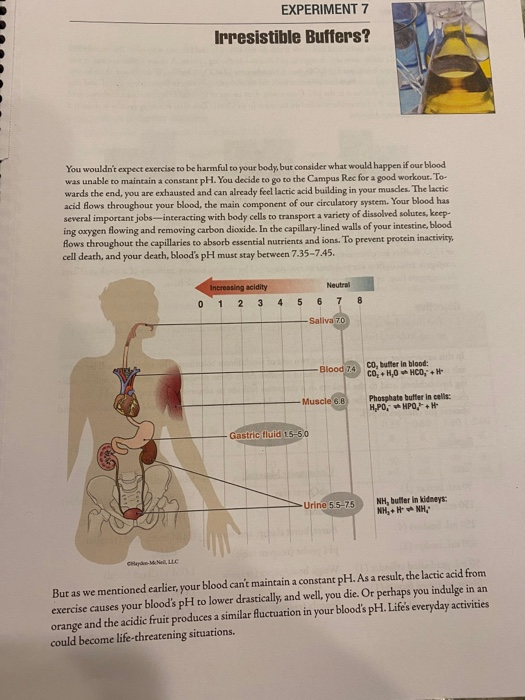Please answer why to #1 and answer #2 I posted the directions so it is easier to understand. EXPERIMENT 7 Irresistible Buffers? You wouldn't expect exercise to be harmful to your body, but consider what would happen if our blood was unable to maintain a constant pH. You decide to go to the Campus Rec for a good workout. To wards the end, you are exhausted and can already feel lactic acid building in your muscles. The lactic acid flows...

• I don't understand what calculations I would have made to create the buffer solution from Part...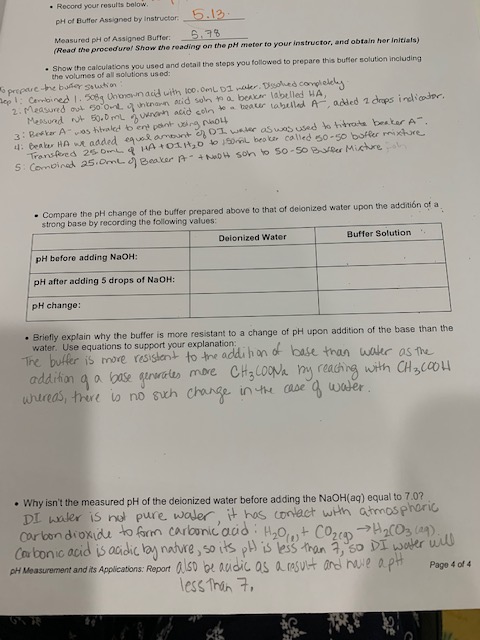I don't understand what calculations I would have made to create the buffer solution from Part D of the lab. I understood the procedures I took to create the solution but when the report asks to show the calculations used to prepare the buffer solution I am not sure other than mixing the volumes indicated in the procedures section. Thank you pH of Butter Assigned by Instructor 5.12. Measured pH of Assigned B r .9 (Read the procedural Show the...

• Please explain Buffers: Solutions That Resist pH Change. Below you will find questions. 1. Define buffer...

Please explain Buffers: Solutions That Resist pH Change. Below you will find questions. 1. Define buffer and know that a buffer typically consists of a weak acid and its conjugate base. 2. Know that the common ion effect is an example of Le Châtelier’s principle. 3. Calculate the pH of a buffer solution starting with initial concentrations of weak acid and its conjugate base. 4. Use the Henderson-Hasselbalch equation to calculate the pH of a buffer solution from the pKa...